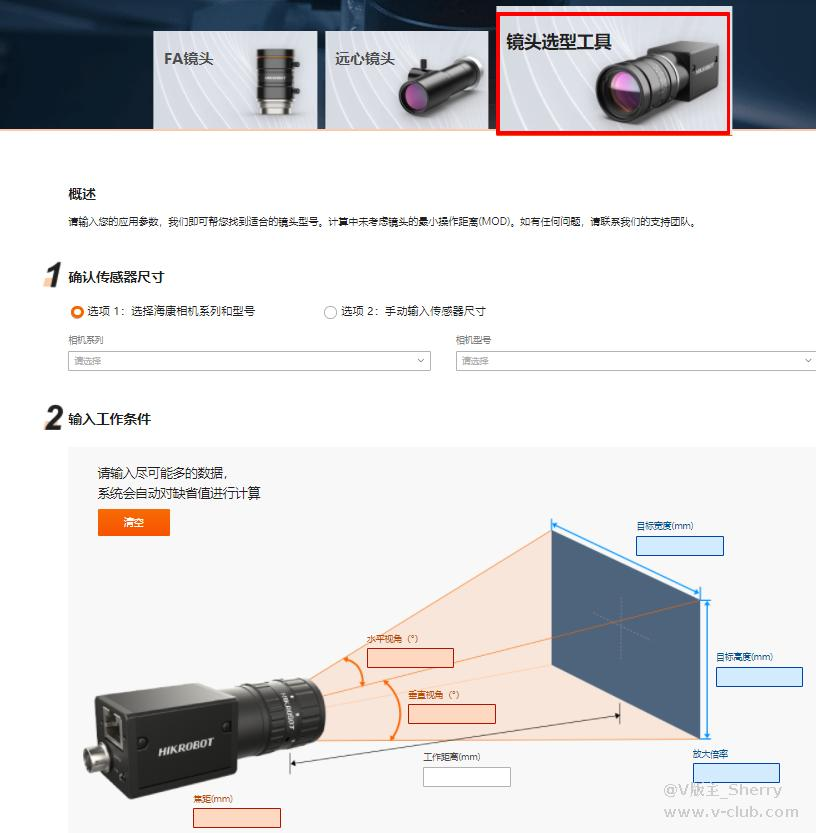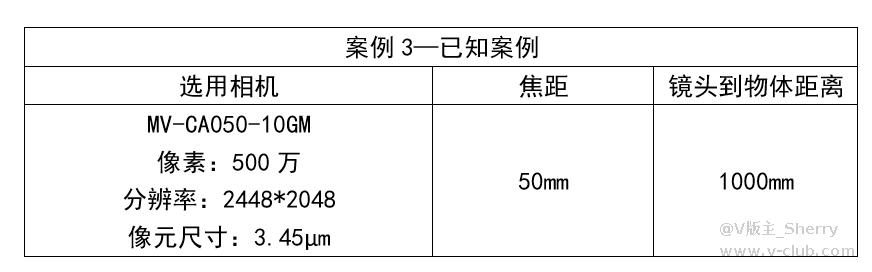## 2.1计算镜头焦距

（1）Sensor长宽尺寸 = (Sensor长度或高度*像元尺寸)/1000可计算出：
Sensor长度 = 2448*3.45/1000 = 8.4456mm
Sensor高度 = 2048*3.45/1000 = 7.0656mm
（2）光学放大倍率（β） = (Sensor长度（h）)/(视野长度（H）)=8.4456/12≈0.7038
（3）焦距（f）= 物距（D）*光学放大倍率（β）= 75*0.7038 =52.785mm，因此可以选择50mm焦距镜头
（4）实际光学放大倍率 = 焦距（f）/物距（D） =50/75≈0.667

（5）单像素精度 = (视野长度（H）)/Sensor长方向有效像素个数=12.662/2448≈0.0052mm
（6）结论：选用50mm镜头，搭配500万像素相机，可以达到12.662mm x 10.593mm的视野，单像素精度5.2µm

###Sensor长度高度：h = 2448 x 3.45µm =8.4456mm, v = 2048 x 3.45µm = 7.0656mm

X = 8.4456 x 1000/50 = 168.91mm, Y = 7.0656 x 1000/50 =141.31 mm

2θ = 2 x arctan(h或v/2/f)
2θx = 2 x arctan(8.4456/2/50) =10° , 2θy = 2 x arctan(7.0656/2/50) = 8°
*工作距离较近时，计算结果与实测结果会有一定的差异，计算数据仅供参考，需要以实测为主。